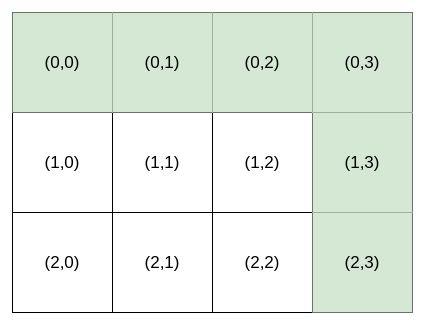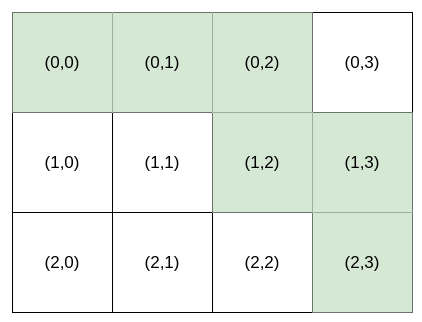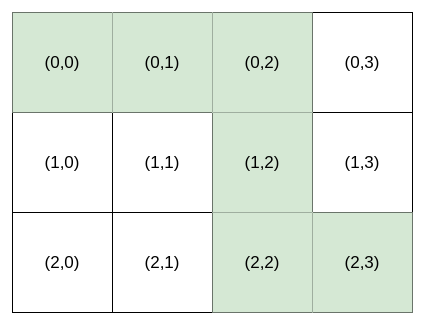1643. Kth Smallest Instructions

Bob is standing at cell `(0, 0)`, and he wants to reach `destination`: `(row, column)`. He can only travel right and down. You are going to help Bob by providing instructions for him to reach `destination`.

The instructions are represented as a string, where each character is either:

• `'H'`, meaning move horizontally (go right), or
• `'V'`, meaning move vertically (go down).

Multiple instructions will lead Bob to `destination`. For example, if `destination` is `(2, 3)`, both `"HHHVV"` and `"HVHVH"` are valid instructions.

However, Bob is very picky. Bob has a lucky number `k`, and he wants the `kth` lexicographically smallest instructions that will lead him to `destination`. `k` is 1-indexed.

Given an integer array `destination` and an integer `k`, return the `kth` lexicographically smallest instructions that will take Bob to `destination`.

Example 1:```Input: destination = [2,3], k = 1
Output: "HHHVV"
Explanation: All the instructions that reach (2, 3) in lexicographic order are as follows:
["HHHVV", "HHVHV", "HHVVH", "HVHHV", "HVHVH", "HVVHH", "VHHHV", "VHHVH", "VHVHH", "VVHHH"].
```

Example 2:```Input: destination = [2,3], k = 2
Output: "HHVHV"
```

Example 3:```Input: destination = [2,3], k = 3
Output: "HHVVH"
```

Constraints:

• `destination.length == 2`
• `1 <= row, column <= 15`
• `1 <= k <= nCr(row + column, row)`, where `nCr(a, b)` denotes `a` choose `b`​​​​​.

1643. Kth Smallest Instructions
``````struct Solution;

impl Solution {
fn kth_smallest_path(destination: Vec<i32>, k: i32) -> String {
let n = (destination + 1) as usize;
let m = (destination + 1) as usize;
let mut res = "".to_string();
let mut dp = vec![vec![0; m]; n];
for i in (0..n).rev() {
for j in (0..m).rev() {
if i == n - 1 && j == m - 1 {
dp[i][j] = 1;
continue;
}
if i == n - 1 {
dp[i][j] = dp[i][j + 1];
continue;
}
if j == m - 1 {
dp[i][j] = dp[i + 1][j];
continue;
}
dp[i][j] = dp[i][j + 1] + dp[i + 1][j];
}
}
Self::build(0, 0, k, &mut res, &dp, n, m);
res
}

fn build(i: usize, j: usize, k: i32, path: &mut String, dp: &[Vec<i32>], n: usize, m: usize) {
if i == n - 1 && j == m - 1 {
return;
}
if i == n - 1 {
path.push('H');
Self::build(i, j + 1, k, path, dp, n, m);
return;
}
if j == m - 1 {
path.push('V');
Self::build(i + 1, j, k, path, dp, n, m);
return;
}
if k <= dp[i][j + 1] {
path.push('H');
Self::build(i, j + 1, k, path, dp, n, m);
} else {
path.push('V');
Self::build(i + 1, j, k - dp[i][j + 1], path, dp, n, m);
}
}
}

#[test]
fn test() {
let destination = vec![2, 3];
let k = 1;
let res = "HHHVV".to_string();
assert_eq!(Solution::kth_smallest_path(destination, k), res);
let destination = vec![2, 3];
let k = 2;
let res = "HHVHV".to_string();
assert_eq!(Solution::kth_smallest_path(destination, k), res);
let destination = vec![2, 3];
let k = 3;
let res = "HHVVH".to_string();
assert_eq!(Solution::kth_smallest_path(destination, k), res);
}
``````